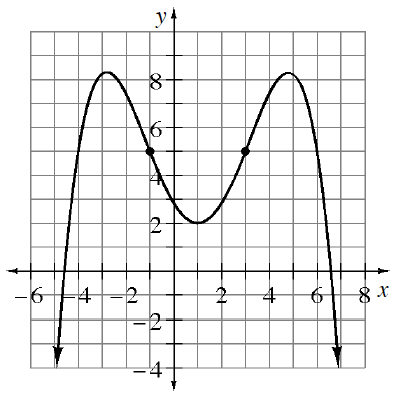### Home > PC3 > Chapter Ch8 > Lesson 8.3.3 > Problem8-119

8-119.

State the intervals where each of the following functions is increasing, decreasing, concave up, and concave down.

1.1. $j ( x ) = \left\{ \begin{array} { c c c } { 2 + 3 x ^ { 2 } } & { \text { if } } & { x \leq 0 } \\ { 2 - x ^ { 2 } } & { \text { if } } & { x > 0 } \end{array} \right.$

Sketch a graph of the function.